## Introduction

Comets are valuable targets for science and exploration missions. It is widely accepted that comets are among the most chemically pristine remnants of the early Solar system, thus allowing glimpses into the chemical and physical properties of the solar nebula at the time and location of their formation. Comets also contain vast amounts of easy-to-access water ice that can be leveraged in future manned and robotic mission architectures through in situ resource utilization (ISRU). In order to access the scientific and economic benefits of comets, one must be able to conduct precise operations near and on their surfaces.

Several comet missions have been flown in the past, though all but one have been flyby missions where the spacecraft was only in the vicinity of the comet for several hours and was never gravitationally bound, e.g., Giotto, Deep Space 1, Deep Impact, EPOXI, Stardust, and Stardust-NExT. To date the only mission to orbit a comet is the Rosetta mission that operated at comet 67P/Churyumov–Gerasimenko (CG) from August 2014 to September 2016 (Accomazzo et al. 2016, 2017). Difficulty in accurately modeling the drag forces resulting from the coma resulted in significant operational costs and effort for Rosetta, where uncertainty in the coma drag required multiple sequences to be developed in tandem. (Bielsa and Herfort 2014; Vallat et al. 2017) Both EPOXI at 103P/ Hartley 2 (A’Hearn et al. 2011; Kelley et al. 2013) and Rosetta at CG (Rotundi et al. 2015; Davidsson et al. 2015; Agarwal et al. 2016) observed gravitationally bound grains orbiting the nucleus. The redistribution of these particles on comets is an important process affecting a comet’s evolution and activity. (A’Hearn et al. 2011; Agarwal et al. 2016; Keller et al. 2017; El-Maarry et al. 2017)

In general, the dynamical environment about an active comet is poorly understood due to large uncertainties in coma and aerodynamic properties, but has significant engineering and science implications. The unbound atmosphere of gas and dust that surrounds a comet is known as the coma. The quantity and behavior of the gases in the coma cannot be predicted accurately and are not known a priori and are, in general, poorly understood without dense datasets. (Mysen et al. 2010; Fougere et al. 2016; Combi et al. 2020)

The most sophisticated coma models to date use discrete simulation Monte Carlo (DSMC) techniques to model gas kinetics, non-spherical nucleus effects, accurate insolation geometry, and even differing activity levels on the surface. (Fougere et al. 2016) These models have nearly infinite degrees of freedom and require careful calibration with real data to produce meaningful results. DSMC models are well suited to a posteriori analysis, but not for operations or prediction. Similar work has been done using computational fluid dynamics (CFD) approaches where the gases are modeled as continuum flow rather than discrete molecules (e.g., Rodionov et al. 2002).

Operationally, spherical harmonics (Scheeres et al. 2000; Bielsa and Herfort 2014) and discrete jet (Byram et al. 2007a) models have been proposed. Recent DSMC simulations suggest that while dust features in comae appear collimated, the gases are not. Gases released from discrete surface sources expand laterally and are not well modeled by discrete jets. (Combi et al. 2012) Note this does not rule out the possibility of collimated gas jets but does suggest that most observed features do not fit this model. While spherical harmonics represent an intuitive way to describe the density field of a cometary coma, analogous to non-spherical gravity fields, there is little predictive framework before arrival. The gas dynamics need not follow a potential function. The spherical harmonic representation of the dynamic pressure field is only a choice of basis function, not a solution to the governing equations. Under the assumption of radial outgassing, any gas distribution should be well modeled to the surface (Scheeres et al. 2000); however, for non-spherical nuclei one can readily expect that the near nucleus gas velocity field will have significant non-radial components that tend toward radial as the distance to the nucleus increases (Fougere et al. 2016; Mysen et al. 2010).

Planetary scientists most often use the Haser model to describe the distribution of gases within the coma. (Combi et al. 2004) The Haser model assumes the coma is spherically symmetric and has a constant outflow velocity and allows for parent species to fragment into daughter species. Neglecting dissociation, this leaves the spherically symmetric outgassing model where the distribution of gases is completely defined by the production rate of each species Q, the distance from the center of the comet r, and the outgassing velocity V. This is also known as a free radial outflow model. Typically, it is assumed that the gas velocity is on the order of 0.5 km/s (Feaga et al. 2007; A’Hearn et al. 2011). These models are useful for remote sensing applications where asymmetries in the coma can be poorly resolved or averaged out. Dissociation becomes important when considering gases that have been exposed to sunlight for times comparable to or longer than their photodissociation lifetimes, typically on the order of one day for water vapor (Combi et al. 2004), at which point they are on the order of 50,000 km from the nucleus. This is well beyond the inner-coma region that this paper is concerned with.

While each of the models discussed above has strengths and weaknesses, none satisfy the needs of this research. Numerical methods such as CFD and DSMC are too complex and case dependent. Spherical harmonics are not convenient representations as the orbit plane is constant under the assumption of radial outgassing and a cannonball spacecraft, resulting in most of the modeled information being unused. Furthermore, spherical harmonic representations are flexible but harder to interpret intuitively. The Haser and free radial outflow models neglect asymmetries in the coma that are important dynamically as shown in this work and others (Scheeres et al. 1998).

In general, for any steady-state coma model assuming purely radial, constant velocity, outgassing, neglecting dissociation and extended sources, it can be easily shown that the density distribution must vary radially as $$1/r^2$$ by integrating the mass flux leaving the comet and holding the production rate and gas velocity constant. Variations with angular position can be expected due to the slow rotation rate of nuclei relative to their thermal inertia (i.e., the nucleus will not, in general, be isothermal), and nucleus shape effects (Groussin et al. 2007). Deviations from a spherically symmetric coma are of interest due to the fact that actual comae are complex (e.g., Combi et al. 2020; Mysen et al. 2010), and introduce additional perturbations to orbits, discussed in detail throughout this paper, necessitating the use of more complex, analytically tractable, models. In this paper, a Fourier series in the argument of latitude modulated by the inverse square of the spacecraft-comet distance is used to model the coma, discussed in detail in Sect. 4.1.

Modeling the drag force acting on a spacecraft is a non-trivial task (Prieto et al. 2014). Gases in planetary upper atmospheres and cometary comae are rarefied and behave differently than the continuum flows found in most traditional terrestrial aerodynamics applications (Bird 1994; Fougere et al. 2016; Prieto et al. 2014). Drag modeling for spacecraft encompasses two broad subfields. The first is how gas particles interact with each other in the flow and how information is transmitted through the flow, if at all (Bird 1994; Prieto et al. 2014). The second is how gas particles interact with the spacecraft (Bird 1994; Prieto et al. 2014; Mehta et al. 2014). DSMC is necessary to model information transmittal in rarefied flows and thus to produce high-fidelity spacecraft drag models; however, this neglects uncertainty in the gas–surface interaction (GSI) model (Bird 1994; Mehta et al. 2014). In the limit that the flow is sufficiently rarefied that gas particles do not interact with each other, drag is dominated entirely by gas–surface interactions (Prieto et al. 2014; Bird 1994). For the remainder of this paper, drag modeling focuses entirely on GSI models and neglects reflected particles or higher-order aerodynamic effects from information being transmitted “upstream.”

Numerical and analytical studies of objects orbiting in close proximity to comets have been conducted. Richter and Keller (1995) investigated the dynamics of long-lived large grains orbiting far from the nucleus. Fulle (1997) numerically found conditions where grains could be injected into these orbits and found that a spherically symmetric coma could not be used for dynamical analyses interested in dust lofting from the surface because any lofted grain would be unbound, which is not realistic. The spherically symmetric coma modifies the effective gravitational parameter of the comet making ejection into bound or unbound orbits trivial to evaluate (Fulle 1997). Scheeres et al. (1998) analytically investigated the effects of a continuous outgassing field and a simplified jet outgassing field on an orbiting spacecraft and found conditions for stable orbits that minimize the effect of outgassing on the orbit. This work has been expanded to higher-fidelity jet models (Byram et al. 2007a; Byram 2009; Byram et al. 2007b). Mysen and Aksnes (2006) performed an orbit averaging analysis using the spherical harmonics coma model presented in (Scheeres et al. 2000) assuming radial drag only. Their analysis showed an asymptotic behavior that drives the semi-major axis and eccentricity to infinity and argument of pericenter to a specific value for terminator orbits where the gas distribution is asymmetric.

The advent of complex numerical coma models has allowed high-fidelity numerical simulations of spacecraft and particles in close proximity to an active comet. For example, Mysen et al. (2010) explored the effect of outgassing on a spacecraft and compared the Doppler signals introduced by coma drag to those of the second degree gravity field, but were not interested in the orbits themselves. Similar studies have been conducted for grains orbiting in the inner coma. Lai et al. (2017) propagated dust trajectories to explore erosion rates across CG. They highlight regions which are more likely to release escaping grains and regions where grains that do not escape are likely to be redeposited.

Though numerical models can be used to propagate trajectories in the coma, assuming gas–surface interaction and coma models, it is difficult to gain general insights from them. In this work, first-order orbit averaging is used to understand the effects of coma drag using simplified coma and drag models. This paper will present semi-analytical equations of motion for the average orbital elements of objects in the coma using generalized models, which will then be applied to simple, yet representative, drag and coma models. Orbits are characterized using Keplerian orbital elements because they are well-known and intuitive. First, simplified coma and drag models are described in the following section (Sect. 2). An overview of first-order orbit averaging is presented in Sect. 3. Next, a Fourier-series expansion of the coma drag perturbation is utilized for a first-order orbit-averaging analysis. These general results are then interpreted using several coma models. Numerical validation is shown in Sect. 5.

## Coma and drag models

### Simplified coma model

Due to the complexity and uncertainty of the gas density and velocity fields in the coma as well as that of the spacecraft drag acceleration, a generalized approach is taken and other perturbing forces such as solar radiation pressure (SRP), non-spherical gravity, and third-body effects are neglected under the assumption that coma drag is the dominant perturbation. The gas density of the coma has been shown through observation and simulation to be consistent, to lowest order, with an inverse-square dependence on distance (e.g., Fink et al. 2016; Moretto et al. 2017). The bulk velocities in the coma also tend to be radial (Mysen et al. 2010), especially as the distance from the comet increases. In this work, it is assumed that the flow velocity is purely radial and is constant throughout the coma. While these models have been framed to represent density variations in the coma, the applicability is broader and can represent the dynamic pressure of the coma, thus accounting for density and velocity variations as a function of latitude and longitude. The velocity is still constrained to be constant as a function of radius.

Drag tends to act in the anti-relative velocity direction for spherical, “cannonball,” spacecraft and more generally for microscopically rough, cold, surfaces regardless of shape (Bird 1994). At comets, the relative velocity vector is dominated by the bulk velocity of the gas, which is on the order of 0.5 km/s, while orbital speeds are on the order of 1 m/s. Neglecting the velocity of the spacecraft in the relative velocity for analytical analyses is therefore acceptable.

#### The skewed coma model

The comet frame is defined as a comet-centered frame whose first axis points from the comet to the sun and whose third axis points along the comet’s rotational pole. For the comet frame to be perfectly defined, this constrains the comet’s obliquity to 0$$^\circ$$ or 180$$^\circ$$. For real comets, this is not usually the case; however, for arbitrary obliquity the rotational pole can be constrained to the $$x-z$$ plane for a reasonable approximation. The obliquity of a body is the angle between its orbital and rotational angular momentum vectors. The comet frame can be defined regardless of the rotation pole, with a singularity occurring when the rotation pole lies along the comet–sun line that will occur twice a comet orbit when the obliquity is 90$$^\circ$$. The obliquity of the comet will only affect the validity of models that are dependent on the rotation of the nucleus, such as the rotation-dependent model discussed in Sect. 2.1.3, but will have no bearing on models such as the solar phase angle model in Sect. 2.1.2. The azimuth angle, $$\theta$$, is defined in the $$x-y$$ plane relative to the comet–sun line in the right handed sense. The elevation angle, $$\delta$$, is defined to be positive in the $$+z$$ direction. A visualization of the comet frame is shown in Fig. 1.

The solar phase angle, $$\gamma$$, is defined as the sun-comet-spacecraft angle. The cosine of the soar phase angle is the dot product of the comet-spacecraft and comet-sun unit vectors and is equivalently the first coordinate of the spacecraft unit vector expressed in the comet frame. This is true regardless of the comet rotation pole.

The comet frame and solar phase angles will be used to construct simple coma models using the form:

\begin{aligned} \rho (\mathbf {r})=\frac{\rho _{0,sym}+\rho _{0,skew}\mathrm {f}(\theta ,\delta )}{r^2} , \end{aligned}
(1)

where r is the radial distance from the comet center of mass, $$\rho _{0,sym}$$ is the density of the symmetric component of the coma at unit distance, and $$\rho _{0,skew}$$ is the density of the skewed component at unit distance along the comet–sun line or, equivalently, above the subsolar point, divided by the value of the skew function evaluated at that point. The skew function, $$\mathrm {f}(\theta ,\delta )$$, is an arbitrary function that describes the gas distribution of the skew component. The model can also be reparameterized using a single unit radius density above the subsolar point, $$\rho _0$$. Note that $$\rho _{0,sym}$$, $$\rho _{0,skew}$$, and $$\rho _0$$ have units of mass per distance because the density is normalized to unit distance. The skewedness of the coma, $$\alpha _{coma}$$, is then defined as the fraction of gas above the subsolar point in the skewed component. This leads to:

\begin{aligned} \rho _{0,skew}= & {} \rho _0 \alpha _{coma} , \end{aligned}
(2)
\begin{aligned} \rho _{0,sym}= & {} \rho _0 (1-\alpha _{coma}) , \end{aligned}
(3)
\begin{aligned} \rho (\mathbf {r})= & {} \rho _0 \frac{(1-\alpha _{coma})+\alpha _{coma}\mathrm {f}(\theta ,\delta )}{r^2} . \end{aligned}
(4)

The skew function, $$\mathrm {f}(\theta ,\delta )$$, should be chosen to yield a realistic, yet simple, coma and should have unit value above the subsolar point. The spherically symmetric component captures uniform outgassing, for example, when the comet is warm enough at night for ices to sublimate, and the skew component captures the dominant effects of insolation. Coma properties are assumed to be independent of time, though real comets will experience random variations in coma properties (i.e., outbursts) as well as variations diurnal, seasonal, orbital, and multi-orbit timescales (e.g., Schleicher 2007; A’Hearn et al. 2005). This form of $$\rho ({\mathbf {r}})$$ is called the skewed coma model.

The activity of a comet is typically described by molecular production rates, $$Q_i$$, where the subscript denotes the $$i_{th}$$ species. In order to pick realistic parameters for the coma models discussed in this paper, it is necessary to relate them to production rates. Production rates for gas species are typically in molecules per second, which can be converted to a mass production rate using the molar mass of the species. By integrating the flux moving through a spherical shell of radius r, the skewed coma model parameters can be related to production rates. In general this yields:

\begin{aligned} Q(r)=\int _{-\pi }^\pi \int _{-\pi /2}^{\pi /2}\rho (\mathbf {r}) {\hat{\mathbf {r}}}\cdot \mathbf {V}_{gas}(\mathbf {r}) r^2 \cos (\delta ) d\delta d\theta . \end{aligned}
(5)

The production rate can be a function of radius. Scenarios for which this can occur include when the coma is time-varying (Combi et al. 2020), the species is a daughter species resulting from the dissociation of other molecules (Combi et al. 2004), when there is an extended source such as sublimating ice grains in the coma (A’Hearn et al. 2011), or when the velocity is not constant with radius.

#### Solar phase angle model

The coma can be modeled assuming that outgassing is dominated by the incident solar flux, which is consistent with the measured low thermal inertia of cometary nuclei (e.g., Groussin et al. 2007; Marshall et al. 2018). A common approach in the literature (Scheeres 2012; Scheeres et al. 1998) is to model the gas distribution in the coma using

\begin{aligned} \mathrm {f}(\theta ,\delta )=\cos (\gamma ), \end{aligned}
(6)

or equivalently in the comet frame:

\begin{aligned} \mathrm {f}(\theta ,\delta )=\cos (\theta )\cos (\delta ). \end{aligned}
(7)

This choice in the skew function limits $$\alpha _{coma}$$ to between 0 and 0.5 inclusive for the model to yield positive densities at all locations in the coma. An analogous parameter relating the production rate to the cosine of the solar incidence angle was estimated using in situ Rosetta mass spectrometer measurements to be 0.98 for water and carbon dioxide and 0.9 for carbon monoxide all in their gaseous form at comet 67P/ Churyumov–Gerasimenko (67P) (Combi et al. 2020). The disparity suggests that realistic comae can be highly skewed beyond what this parameterization allows.

#### Rotation-dependent model

If the comet is assumed to have 0$$^\circ$$ or 180$$^\circ$$ obliquity and some low, but nonzero, thermal inertia, thus allowing some memory of the nucleus’ thermal history, a skew function with a maximum above the subsolar point and local minima above the anti-solar point and at the poles is justified. A function satisfying these constraints is:

\begin{aligned} \mathrm {f}(\theta ,\delta )=\frac{1+\cos (\theta )}{2}\cos (\delta ). \end{aligned}
(8)

Note this model will not reflect the dominant features of the gas distribution as the rotational axis nears the orbital plane or when a large thermal inertia shifts the maximum outgassing point off the subsolar point.

The rotation-dependent coma model is thus:

\begin{aligned} \rho (\mathbf {r})=\frac{\rho _{0,sym}+\rho _{0,skew}\frac{1+\cos (\theta )}{2}\cos (\delta )}{r^2}=\rho _0 \frac{(1-\alpha _{coma})+\alpha _{coma}\frac{1+\cos (\theta )}{2}\cos (\delta )}{r^2} . \end{aligned}
(9)

The coma model must be related to the production rate in order for realistic parameter values to be chosen. Substituting into the production rate integral:

\begin{aligned} Q(r)= & {} \int _{-\pi }^\pi \int _{-\pi /2}^{\pi /2}\rho _0 \frac{(1-\alpha _{coma})+\alpha _{coma}\frac{1+\cos (\theta )}{2}\cos (\delta )}{r^2} \,\,{\hat{\mathbf {r}}}\cdot \mathbf {V}_{gas} r^2 \cos (\delta ) d\delta d\theta , \end{aligned}
(10)
\begin{aligned} Q= & {} \int _{-\pi }^\pi \int _{-\pi /2}^{\pi /2}\rho _0 [(1-\alpha _{coma})+\alpha _{coma}\frac{1+\cos (\theta )}{2}\cos (\delta )]V_{flow} \cos (\delta ) d\delta d\theta , \end{aligned}
(11)

evaluating and rearranging, it is found that:

\begin{aligned} Q= & {} \frac{1}{2} \pi ((\pi -8) \alpha _{coma} +8) V_{flow} \rho _0 , \end{aligned}
(12)
\begin{aligned} \rho _0= & {} \frac{2 Q}{\pi V_{flow} (8+(\pi -8) \alpha _{coma}) } . \end{aligned}
(13)

Note that these equations require Q and $$\rho _0$$ in commensurate units, so if Q is given in molecules per second, then $$\rho _0$$ will be in molecules per unit volume.

#### Jet model

While continuous outgassing models have grown in favor for modeling the gas distribution in the coma (Kramer et al. 2018; Combi et al. 2012), discrete jet models cannot be ruled out and indeed may be appropriate at some comets and for modeling some outbursts. Discrete jet coma models have been presented in detail (Byram et al. 2007b; Byram 2009). Simplified, impulsive, jet models have been described in (Scheeres 2012). While true jets have dimension, the impulsive assumption is more tractable and produces similar results in most cases (Scheeres 2012).

For this work, the impulsive jet model is adopted as:

\begin{aligned} \rho (\mathbf {r})= \frac{\rho _0}{r^2}\delta _D(\beta ) , \end{aligned}
(14)

where $$\delta _D(x)$$ is the Dirac Delta function and $$\beta$$ is the spacecraft–comet–jet angle. This is a good approximation of the effect of jets as long as the spacecraft only interacts with the jet for a small fraction of its orbit. Note that here, $$\rho (\mathbf {r})$$ is infinite when the spacecraft passes through the jet and is zero elsewhere, but the density and production rate can still be related using Eq. (5).

### Simplified drag model

In this work, the spacecraft/natural object is modeled as a sphere such that the only aerodynamic force acting on the spacecraft is drag. As discussed in Sect. 1, it is assumed that the flow is rarefied and thus that momentum is transferred to the spacecraft from the flow through collisions and that no information is passed upstream. A simplified Maxwellian drag model (Bielsa and Herfort 2014) is adopted; however, for the purpose of this research the accommodation coefficient, spacecraft temperature, and the fraction of elastic and inelastic collisions are absorbed into the drag coefficient. This results in:

\begin{aligned} \mathbf {F}_{drag}= & {} -\frac{1}{2}\rho C_d s V^2_{rel}\hat{{\mathbf {V}}}_{rel} , \end{aligned}
(15)
\begin{aligned} \mathbf {V}_{rel}= & {} \mathbf {V}_{sc}-\mathbf {V}_{gas} , \end{aligned}
(16)

where $$\mathbf {F}_{drag}$$ is the drag force, $$\rho$$ is the local flow density, $$C_d$$ is the drag coefficient, s is the object reference area, $$\mathbf {V}_{sc}$$ is the inertial spacecraft velocity, and $$\mathbf {V}_{gas}$$ is the inertial flow velocity. While this equation is identical to its terrestrial counterpart, which may seem counterintuitive, it can be derived from conservation of momentum (Bielsa and Herfort 2014). Note that for perfectly elastic collisions $$C_d$$ is 2, while for perfectly inelastic collisions $$C_d$$ is 1. The inclusion of emission of absorbed gas molecules or the thermal accommodation of molecules with the spacecraft during collisions allows $$C_d$$ to rise above 2. A $$C_d$$ of 2.2 is often assumed, (Vallado and McClain 2001) as will be adopted here. If the object had a more complex shape, then the drag force would not necessarily be constrained to the anti-relative velocity direction.

For numerical simulation, the relative velocity is used; however, for orbit averaging the relative velocity can be approximated as the gas velocity resulting in:

\begin{aligned} \mathbf {a}_{drag}=\frac{1}{2}\rho C_d \dfrac{s}{m} V^2_{gas}{\hat{\mathbf {r}}} . \end{aligned}
(17)

## Orbit averaging theory

In this paper, Gauss’ planetary equations are orbit-averaged and used to gain analytical insights into the effect of coma drag on a spacecraft or particle near a comet. Numerical simulations of a spacecraft in a cometary coma will be conducted. Finally, semi-analytical solutions to the orbit-averaged differential equations for the elements will be compared to numerical solutions. The orbit-averaged orbital elements are referred to as the mean elements throughout this work without ambiguity in the averaging period.

This work considers only two forces acting on a spacecraft of mass m: two-body gravity between the comet and spacecraft, and coma drag. It is assumed that the mass of the spacecraft is much less than the mass of the comet and thus can be neglected. Any accelerations from the comet frame accelerating as the comet orbits the sun are neglected as it is assumed that they are small and for the sake of generality. This paper is interested in the effects induced by drag; thus, all other perturbations are neglected. Furthermore, any additional sufficiently small perturbations can be added linearly. The equations of motion are thus defined as:

\begin{aligned} \mathbf {a}_{sc}=-\frac{\mu }{r^3}\mathbf {r}+\mathbf {a}_{drag} , \end{aligned}
(18)

where $$\mathbf {a}_{sc}$$ is the inertial acceleration of the spacecraft in the comet frame and $$\mu$$ is the gravitational parameter of the comet.

While conditions in the coma vary on diurnal, seasonal, and orbital timescales, it is assumed that they are constant in the comet frame over the time of the simulation. Other perturbations such as third-body effects, solar radiation pressure, and non-spherical gravity are neglected in this analysis, though they can also be important at a comet. Future papers will explore the complex dynamics associated with a time-varying coma with more complex gas density and velocity variations and conduct high-fidelity simulations with more complete dynamics. The purpose of this analysis is to investigate how coma drag perturbs the dynamics, not to conduct high-fidelity simulations representative of a specific situation. The Hill radius provides an indication of where the comet’s gravity is dominant over third-body effects from the Sun. For CG, the Hill radius is 220 km at pericenter and 1010 km at apocenter. When a spacecraft is very close to the nucleus, within several nuclear radii, non-spherical gravity and non-radial components of the coma flow velocity can become important. The dynamics assumed here are valid well inside the Hill radius and more than a few nuclear radii from the comet. Note that for well-characterized perturbations where first-order averaging can be applied, such as SRP, the averaged effects of the perturbations can be added linearly and do not need to be averaged simultaneously.

Lagrange’s and Gauss’ planetary equations describe the effect of perturbing forces on the Keplerian orbital elements for conservative and general forces, respectively (Vallado and McClain 2001). Because drag is a non-conservative force, even neglecting spacecraft velocity, it cannot be described using a potential function. This necessitates the use of Gauss’ form of the planetary equations for the averaging analysis.

Gauss’ planetary equations are typically formulated as follows (Vallado and McClain 2001):

\begin{aligned} \frac{d i}{d t}= & {} \frac{r \cos (\theta ^*)}{n a^2 \sqrt{1-e^2}}a_h , \end{aligned}
(19)
\begin{aligned} \frac{d \varOmega }{d t}= & {} \frac{r \sin (\theta ^*)}{n a^2 \sqrt{1-e^2} \sin (i)}a_h , \end{aligned}
(20)
\begin{aligned} \frac{d \omega }{d t}= & {} \frac{\sqrt{1-e^2}}{n a e}\left[ -\cos (\nu ) a_r + \sin (\nu )\left( 1+\frac{r}{p}\right) a_\theta \right] -\frac{r\cos (i)\sin (\theta ^*)}{h \sin (i)}a_h , \end{aligned}
(21)
\begin{aligned} \frac{d a}{d t}= & {} \frac{2}{n \sqrt{1-e^2}}\left[ e \sin (\nu ) a_r + \frac{p}{r}a_\theta \right] , \end{aligned}
(22)
\begin{aligned} \frac{d e}{d t}= & {} \frac{\sqrt{1-e^2}}{n a}\left[ \sin (\nu )a_r+\left( \cos (\nu )+\frac{e+\cos (\nu )}{1+e \cos (\nu )}\right) a_\theta \right] , \end{aligned}
(23)
\begin{aligned} n= & {} \sqrt{\frac{\mu }{a^3}} , \end{aligned}
(24)
\begin{aligned} p= & {} a (1-e^2) , \end{aligned}
(25)
\begin{aligned} h= & {} \sqrt{\mu p} , \end{aligned}
(26)
\begin{aligned} \theta ^*= & {} \nu +\omega , \end{aligned}
(27)

where a is the semi-major axis, e is the eccentricity, i is the inclination, $$\varOmega$$ is the right ascension of the ascending node, and $$\omega$$ is the argument of pericenter of the orbit, with $$\nu$$ being the true anomaly, all as defined in the Keplerian sense. The mean motion, n, semi-latus rectum, p, scalar angular momentum, h, and argument of latitude, $$\theta ^*$$, are also defined in the Keplerian sense. In Gauss’ planetary equations, the perturbing acceleration is expressed in the radial, in-track, cross-track frame (RIC), where the radial vector is defined by the position of the spacecraft relative to the center of mass of the comet and the cross-track vector is parallel to the orbital angular momentum vector of the spacecraft, and where the in-track vector completes the right-handed triad. The perturbing acceleration vector has components $$a_r$$, $$a_\theta$$, and $$a_h$$, in the radial, in-track, and cross-track, directions, respectively.

Because this analysis is concerned with orbit averaged effects, it is not necessary to include dM/dt or $$d M_0/d t$$ in this analysis. By averaging over one orbit, it is possible to isolate long-term trends from periodic variations, thus tracking the shape and orientation of the orbit, but not the anomaly of the object within that orbit. It is assumed that the orbital elements are constant over one orbit in a first-order averaging analysis. In other words, the average orbital elements change sufficiently slowly compared to the orbital period.

Differential equations for the orbit-averaged elements can be found using the following methodology: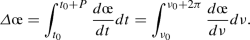(28)

Because Gauss’ equations are time derivatives, they must be converted from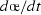to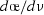by using the second-order Sundman transformation, or equivalently Kepler’s second law expressed in polar coordinates:

\begin{aligned} \frac{d \nu }{d t}=\frac{h}{r^2} . \end{aligned}
(29)

Using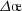, the differential equations for the averaged orbital elements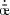can be derived by dividing by the instantaneous Keplerian orbital period P: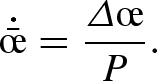(30)

## Orbit averaging analysis

In this section, differential equations for the mean elements are derived for the radial inverse-square Fourier series perturbing acceleration. These equations are explored in detail. The results are then used to analyze the phase-angle, rotation-dependent, and discrete jet coma models. Comparisons with previous derivations are presented.

### Fourier series representation

Taking advantage of the general coma properties discussed in Sect. 2.1 and that the orbital plane is unperturbed by radial perturbations, the perturbing coma drag acceleration can be generically expressed as a Fourier series in terms of the argument of latitude, which is the sum of the true anomaly, $$\nu$$, and the argument of pericenter $$\omega$$ in an inertial frame:

\begin{aligned} a_r=\frac{1}{r^2}\sum \limits _{m=0}^\infty A_m \cos (m (\nu +\omega ))+B_m \sin (m (\nu +\omega )) , \end{aligned}
(31)

which can be expanded using the trigonometric sum identities:

\begin{aligned}&a_r=\frac{1}{r^2}\sum \limits _{m=0}^\infty A_m \cos (m\nu )\cos (m\omega )-A_m \sin (m\nu )\sin (m\omega )\nonumber \\&\qquad +B_m \sin (m\nu )\cos (m\omega )+ B_m \cos (m\nu )\sin (m\omega ). \end{aligned}
(32)

Collapsing the Fourier series using the shorthand:

\begin{aligned} a_r=\frac{1}{r^2}\sum \limits _{m=0}^\infty f_m(\nu , \omega ) , \end{aligned}
(33)

where $$a_r$$ is the radial drag acceleration, $$A_m$$ and $$B_m$$ are the $$m^{th}$$-order Fourier coefficients, and r is the scalar distance to the comet. In the interest of generality, it is useful to explicitly state that for $$a_r$$ to represent coma drag, the perturbing acceleration must be positive for all $$\nu$$ and $$\omega$$; however, the averaging analysis is valid for any inverse-square radial force regardless of sign.

Noting that the perturbing acceleration is only in the radial direction, converting Gauss’ equations fromto, and substituting Eq. (33) into Gauss’ equations:

\begin{aligned} \frac{d i}{d\nu }= & {} \frac{d \varOmega }{d\nu }=0 , \end{aligned}
(34)
\begin{aligned} \frac{d \omega }{d\nu }= & {} -\cos (\nu )\frac{1}{n a e\sqrt{\mu a}}\sum \limits _{m=0}^\infty f_m(\nu , \omega ) , \end{aligned}
(35)
\begin{aligned} \frac{d a}{d\nu }= & {} \sin (\nu )\frac{2 e}{n (1-e^2) \sqrt{\mu a }}\sum \limits _{m=0}^\infty f_m(\nu , \omega ) , \end{aligned}
(36)
\begin{aligned} \frac{d e}{d\nu }= & {} \sin (\nu )\frac{1}{n a \sqrt{\mu a}}\sum \limits _{m=0}^\infty f_m(\nu , \omega ) . \end{aligned}
(37)

For first-order averaging the elements, except for true anomaly, are held constant over one orbit (recall Eq. (28)). Recognizing that $$\sin (x)$$ and $$\cos (x)$$ are orthogonal functions, as are $$\sin (mx)$$ and $$\cos (mx)$$ where m is an integer greater than or equal to 0, over the interval $$[0,2\pi ]$$, evaluatingbecomes simple as all but the first-order terms drop out of the averaging integral:

\begin{aligned} \varDelta i= & {} \varDelta \varOmega =0 , \end{aligned}
(38)
\begin{aligned} \varDelta \omega= & {} -\pi \frac{A_1 \cos (\omega )+B_1 \sin (\omega )}{n a e\sqrt{\mu a}} , \end{aligned}
(39)
\begin{aligned} \varDelta a= & {} \pi \frac{2 e \left[ -A_1 \sin (\omega )+B_1 \cos (\omega )\right] }{n (1-e^2) \sqrt{\mu a }} , \end{aligned}
(40)
\begin{aligned} \varDelta e= & {} \pi \frac{-A_1 \sin (\omega )+B_1 \cos (\omega )}{n a \sqrt{\mu a}}. \end{aligned}
(41)

These effects are a direct result of the skewedness of the coma, deviation of the coma from spherically symmetric, and are referred to as “skewedness effects.” Using the Keplerian orbital period:

\begin{aligned} P=2\pi /n , \end{aligned}
(42)

and Eq. (30) to find:

\begin{aligned} \dot{{\bar{i}}}= & {} \dot{\bar{\varOmega }}=0 , \end{aligned}
(43)
\begin{aligned} \dot{\bar{\omega }}= & {} -\frac{A_1 \cos (\omega )+B_1 \sin (\omega )}{2 a e\sqrt{\mu a}} , \end{aligned}
(44)
\begin{aligned} \dot{{\bar{a}}}= & {} \frac{ e \left[ -A_1 \sin (\omega )+B_1 \cos (\omega )\right] }{(1-e^2) \sqrt{\mu a }} ,\end{aligned}
(45)
\begin{aligned} \dot{{\bar{e}}}= & {} \frac{-A_1 \sin (\omega )+B_1 \cos (\omega )}{2 a \sqrt{\mu a}} . \end{aligned}
(46)

At this point, it is prudent to consider that the zeroth-order term of the Fourier series, $$A_0$$, acts to modify the gravitational parameter directly, which also changes the angular momentum and energy of the system. Taking the scalar radial acceleration with only a zeroth-order perturbation results in:

\begin{aligned} a_{r,0}=-\frac{\mu }{r^2}+\frac{A_0}{r^2}=-\frac{\mu -A_0}{r^2} , \end{aligned}
(47)

where it becomes clear that the equivalent gravitational parameter for this system is:

\begin{aligned} \mu _{eq}=\mu -A_0 . \end{aligned}
(48)

Note that for coma drag $$A_0$$ must greater than 0 in the non-trivial case; however, any radial, inverse-square, perturbing acceleration can be analyzed in this way and need not be outward. The complete differential equations for the averaged orbital elements are:

\begin{aligned} \dot{{\bar{i}}}= & {} \dot{\bar{\varOmega }}=0 , \end{aligned}
(49)
\begin{aligned} \dot{\bar{\omega }}= & {} -\frac{A_1 \cos (\omega )+B_1 \sin (\omega )}{2 a e\sqrt{(\mu -A_0) a}} , \end{aligned}
(50)
\begin{aligned} \dot{{\bar{a}}}= & {} \frac{ e \left[ -A_1 \sin (\omega )+B_1 \cos (\omega )\right] }{(1-e^2) \sqrt{(\mu -A_0) a }} , \end{aligned}
(51)
\begin{aligned} \dot{{\bar{e}}}= & {} \frac{-A_1 \sin (\omega )+B_1 \cos (\omega )}{2 a \sqrt{(\mu -A_0) a}} . \end{aligned}
(52)

It is necessary to use the modified gravitational parameter to calculate and propagate the orbital elements in this perturbed system as there is no physical difference between a change in gravitational parameter and a change in $$A_0$$. The equivalent gravitational parameter must be used to calculate the period for numerical averaging of the osculating orbital elements. Note that these equations are valid only for bound orbits with an eccentricity between 0 and 1 non-inclusive.

#### Interpretations and equilibria

Several fundamental properties of the orbit evolution can be found through inspection of Eqs. (49) to (52). Trivially, one can conclude that the orbit plane remains constant. Perhaps the most surprising result is that the average elements are only effected by the zeroth- and first-order terms of the Fourier expansion of the perturbing acceleration for an arbitrary orbit. This implies that the modeling of coma drag and other inverse-square radial forces, such as thermal radiation pressure, need not be high fidelity to analyze the averaged effect on an orbiting spacecraft or particle. Similar results have been observed for the binary YORP effect resulting SRP acting on binary asteroids that are asymmetric and synchronously rotating, though only for near circular orbits (McMahon and Scheeres 2010).

The signs of the differential equations for the average orbital elements are entirely governed by the argument of pericenter for a given physical system. Furthermore, $$\dot{\bar{\omega }}$$ is orthogonal to $$\dot{{\bar{a}}}$$ and $$\dot{{\bar{e}}}$$. For a given orbit, when the magnitude of $$\dot{\bar{\omega }}$$ is maximized then there is no average change in the semi-major axis or eccentricity but when the magnitude of $$\dot{{\bar{a}}}$$ and $$\dot{{\bar{e}}}$$ are maximized, there is no average change in the argument of pericenter. Thus, there no frozen orbits, orbits where all of the orbital elements on average remain constant, for any combinations of $$A_1$$ and $$B_1$$ except the trivial case where $$A_1=B_1=0$$.

While there are no frozen orbits in general, there are “equilibrium” arguments of pericenter for constant arguments of pericenter or semi-major axis and eccentricity. Setting $$\dot{\bar{\omega }}$$ equal to zero requires that:

\begin{aligned} A_1 \cos (\omega _{eq,\omega })+B_1 \sin (\omega _{eq,\omega })=0 , \end{aligned}
(53)

where $$\omega _{eq,\omega }$$ is an equilibrium argument of pericenter. Technically, this should be referred to as the equilibrium argument of pericenter for the argument of pericenter but for reasons to be explained shortly and simplicity $$\omega _{eq,\omega }$$ is referred to as equilibrium argument of pericenter. Solving Eq. (53) yields:

\begin{aligned} \omega _{eq,\omega }=-\tan ^{-1}\left( \frac{A_1}{B_1}\right) , \end{aligned}
(54)

which has 2 solutions on 0 to $$2 \pi$$. For ease of discussion, the solution to a generic inverse tangent that has $$\cos (x)>0$$ is referred to as $$x_+$$, while the solution with $$\cos (x)<0$$ is referred to as $$x_-$$. For completeness, when $$\cos (x)=0$$, the same standard is then applied to $$\sin (x)$$, which equals identically $$\pm 1$$, in that special case.

Setting $$\dot{{\bar{a}}}$$ and $$\dot{{\bar{e}}}$$ equal to zero requires that:

\begin{aligned}&-A_1 \sin (\omega _{eq,a,e})+B_1 \cos (\omega _{eq,a,e})=0 , \end{aligned}
(55)
\begin{aligned}&\omega _{eq, a, e}=\tan ^{-1}\left( \frac{A_1}{B_1}\right) . \end{aligned}
(56)

This result should not be surprising in light of the orthogonality between $$\dot{\bar{\omega }}$$ and $$\dot{{\bar{a}}}$$ or $$\dot{{\bar{e}}}$$. While mathematically equilibrium arguments of pericenter exist for semi-major axis and eccentricity, they are not attainable in that when $$\dot{{\bar{a}}}$$ and $$\dot{{\bar{e}}}$$ are zero, $$\dot{\bar{\omega }}$$ is maximized, thus immediately inducing changes in semi-major axis and eccentricity. For this reason, $$\omega _{eq, a, e}$$ are not discussed further and $$\omega _{eq, \omega }$$ are referred to as the equilibrium arguments of pericenter.

Semi-major axis and eccentricity evolve together as they are both dependent on the same function of $$A_1$$, $$B_1$$, and $$\omega$$. When semi-major axis increases, so does eccentricity and vice versa. This implies that the radius of pericenter, $$r_p$$, cannot evolve independently of a or e. Using

\begin{aligned} \dot{\bar{r_p}}=\dot{{\bar{a}}}(1-e)-a\dot{{\bar{e}}}, \end{aligned}
(57)

the following expression is derived:

\begin{aligned} \dot{\bar{r_p}}= -\frac{(1-e)[-A_1 \sin (\omega )+B_1 \cos (\omega )]}{2 (1+e) \sqrt{(\mu -A_0) a}} . \end{aligned}
(58)

This equation indicates that $$r_p$$ decreases as a and e increase or that $$r_p$$ increases as a and e decrease. While this may seem counter intuitive, it is simply a result that eccentricity change dominates, so while the size of an orbit may be getting larger, its pericenter distance will decrease. An important result of this is that certain arguments of pericenter will increase radius of pericenter, while others will decrease radius of pericenter, providing “safe” and “unsafe” orbit orientations for spacecraft operating at an active comet.

Unfortunately for spacecraft operators, the safe orientation that increases radius of pericenter also decreases semi-major axis and eccentricity. This tends to circularize the orbit until the argument of pericenter can shift to the equilibrium corresponding to increasing semi-major axis and eccentricity, which decreases radius of pericenter. The time rate of change of the argument of pericenter is dependent on 1/e and moves rapidly as the eccentricity of the orbit decreases. Perturbations to the system will prevent the orbit from becoming perfectly circular, and thus, the orbit will not remain at circular because of the strong sensitivity of the argument of pericenter. The equilibrium argument of pericenter that decreases semi-major axis and eccentricity is thus referred to as the unstable argument of pericenter, while the argument that increases semi-major axis and eccentricity is called the stable argument of pericenter. The unstable and stable labels do not imply any linearized stability characteristics, rather they characterize the nonlinear tendencies of the system where orbits will all converge to the stable equilibrium argument of pericenter, even if starting perfectly on the unstable equilibrium.

Note that the unstable equilibrium argument of pericenter is the asymptotic behavior described by Mysen and Aksnes (2006). While they describe the asymptotic behavior using only the first-degree spherical harmonics, it follows from this analysis that the behavior is dependent on the sum of the first-order Fourier coefficients of all degrees of the spherical harmonics expansion. One can also state that the asymptotic behavior found by Mysen and Aksnes (2006) for terminator orbits will also be observed at all orbit plane orientations, whose first-order Fourier coefficients are nonzero.

One can also consider using these averaged dynamics to control the orbit of a spacecraft about a comet, if the coefficients $$A_0$$, $$A_1$$, and $$B_1$$ are known, perhaps by modulating the nadir pointing area of the spacecraft. This analysis shows that if the modulations repeat each orbit based on the argument of latitude, the orbit is not fully controllable and is constrained by Eqs. (49)–(52); however, one can imagine modulating the nadir pointing area to null out $$A_1$$ and $$B_1$$, thus making any orbit frozen regardless of the gas distribution.

While the perturbed orbit on the whole evolves, one can search beyond the Keplerian orbital elements for preserved quantities. For instance, as should be expected for a radial perturbing acceleration, the angular momentum of the orbit remains unchanged and thus so does the semi-latus rectum, p. This can be proved from the differential equations for the averaged orbital elements by using

\begin{aligned} \dot{{\bar{p}}}=\dot{{\bar{a}}}(1-e^2)-2ae\dot{{\bar{e}}} , \end{aligned}
(59)

where substitution for the time derivatives and simplification yields:

\begin{aligned} \dot{{\bar{p}}}=0 , \end{aligned}
(60)

as expected.

The orbit evolution can be searched for points that remain on the orbit in inertial space. To do this, it is convenient to use the nodal frame, with the first axis as the ascending node and the third axis being the orbital angular momentum vector. The nodal frame is inertial under radial perturbations. The radius of crossing, $$r_c$$, can be defined as the radial distance(s) that are constant at specific polar angles, their respective arguments of crossing, an. The argument of crossing is measured prograde relative to the reference direction, in this case the ascending node, and is a specific argument of latitude. The “of crossing” nomenclature is used because the spacecraft will cross through an angular position, the argument of crossing, at a specific radius, the radius of crossing, each orbit. Recognizing that $$an-\omega$$ is a true anomaly, the conic equation can be used to write the radius of crossing as:

\begin{aligned} r_c(\nu )=\frac{a(\nu ) \left( 1-e(\nu )^2\right) }{1+e(\nu ) \cos (an(A_m,B_m)-\omega (\nu ))} , \end{aligned}
(61)

where $$an(A_m,B_m)$$ is the argument of crossing. Differentiating $$r_c$$ with respect to true anomaly, where primes represent derivatives with respect to true anomaly, and inserting Gauss equations and simplifying:

\begin{aligned}&\dfrac{\partial r_c}{\partial \nu }=\frac{\left( 1-e(\nu )^2\right) a'(\nu )}{e(\nu ) \cos (an(A_m,B_m)-\omega (\nu ))+1}-\frac{2 a(\nu ) e(\nu ) e'(\nu )}{e(\nu ) \cos (an(A_m,B_m)-\omega (\nu ))+1} \end{aligned}
(62)
\begin{aligned}&-\frac{a(\nu ) \left( 1-e(\nu )^2\right) \left( e'(\nu ) \cos (an(A_m,B_m)-\omega (\nu ))+e(\nu ) \omega '(\nu ) \sin (an(A_m,B_m)-\omega (\nu ))\right) }{(e(\nu ) \cos (an(A_m,B_m)-\omega (\nu ))+1)^2} ,\nonumber \\&\dfrac{\partial r_c}{\partial \nu }=\frac{a \left( e^2-1\right) (A_m \cos (m(\nu +\omega ) )+B_m \sin (m(\nu +\omega ))) \sin (-an(A_m,B_m)+\nu +\omega )}{\mu (e \cos (\omega -an(A_m,B_m))+1)^2} . \end{aligned}
(63)

Integrating from 0 to 2 $$\pi$$:

\begin{aligned}&\varDelta r_c(m=1)=\frac{\pi a \left( e^2-1\right) (B_1 \cos (an(A_1,B_1))-A_1 \sin (an(A_1,B_1)))}{\mu (e \cos (\omega -an(A_1,B_1))+1)^2} , \end{aligned}
(64)
\begin{aligned}&\varDelta r_c(m\ne 1)=0 . \end{aligned}
(65)

This is consistent with only first-order terms perturbing the orbit on average. Setting Eq. (64) equal to zero and solving for $$an(A_1,B_1)$$ yields:

\begin{aligned}&B_1 \cos (an(A_1,B_1))=A_1 \sin (an(A_1,B_1)) , \end{aligned}
(66)
\begin{aligned}&an(A_1,B_1)= \tan ^{-1} \left( \frac{B_1}{A_1}\right) , \end{aligned}
(67)

where, using the inverse tangent notation discussed above, both the plus and minus tangent solutions are admissible. Recall that this is the same form as the equilibrium arguments of pericenter for semi-major axis and eccentricity and is orthogonal to the equilibrium arguments of pericenter. Therefore, the radius of crossing reduces to the semi-latus rectum when the argument of pericenter is along an equilibrium! While the arguments of crossing can be substituted into Eq. (61) to find the radii of crossing, in general this is sensitive to the osculating elements and thus the analytic form has significant error while the angle is computed exactly. Note that the radius of crossing is constant as shown numerically in Sect. 5; however, it is offset from the predicted value and could theoretically be computed using Gauss’ equations and integrating an anomaly forward to that point from the osculating orbital elements at a reference time.

### Expressing coma models in terms of orbital elements

Each of the coma models (skew functions) described in Sect. 2.1 is expressed in terms of latitude, longitude, or the solar phase angle. In order to perform an averaging analysis, these angles must be expressed in terms of the Keplerian orbital elements. The radius is readily defined by the conic equation:

\begin{aligned} r=\dfrac{a(1-e^2)}{1+e\cos (\nu )} . \end{aligned}
(68)

The position vector can be expressed in the comet frame as:

\begin{aligned} \mathbf {r}_{\mathbf {CF}}=r \begin{bmatrix} \cos (\theta )\cos (\delta )\\ \sin (\theta )\cos (\delta )\\ \sin (\delta )\\ \end{bmatrix} . \end{aligned}
(69)

Using well-known expressions for the position vector in the perifocal frame and the rotation matrix to convert perifocal frame coordinates to comet frame (inertial) coordinates, one can derive the position vector of the spacecraft in the comet frame in terms of r, $$\nu$$, $$\omega$$, $$\varOmega$$, and i:

\begin{aligned} \mathbf {r_{CF}}=r \begin{bmatrix} \cos (\varOmega )\cos (\omega +\nu )-\sin (\varOmega )\cos (i)\sin (\omega +\nu )\\ \sin (\varOmega )\cos (\omega +\nu )+\cos (\varOmega )\cos (i)\sin (\omega +\nu )\\ \sin (i)\sin (\omega +\nu )\\ \end{bmatrix}=\begin{bmatrix} X\\ Y\\ Z\\ \end{bmatrix} . \end{aligned}
(70)

From geometry, it is known that:

\begin{aligned} \delta =\sin ^{-1}(Z/r) , \end{aligned}
(71)

where in this case r is defined by the conic equation. Thus, the elevation angle:

\begin{aligned} \delta =\sin ^{-1}(\sin (i)\sin (\omega +\nu )) . \end{aligned}
(72)

In the context of the coma model, this yields the useful expression:

\begin{aligned} \cos (\delta )=\sqrt{1-\sin ^2(i)\sin ^2(\omega +\nu )} . \end{aligned}
(73)

The azimuth angle, $$\theta$$, can be defined geometrically as:

\begin{aligned} \theta =\cos ^{-1}\left( \dfrac{X}{r\cos (\delta )}\right) . \end{aligned}
(74)

This results in an expression for the azimuth angle:

\begin{aligned} \theta =\cos ^{-1}\left( \dfrac{\cos (\varOmega )\cos (\omega +\nu )-\sin (\varOmega )\cos (i)\sin (\omega +\nu )}{\sqrt{1-\sin ^2(i)\sin ^2(\omega +\nu )}}\right) . \end{aligned}
(75)

The cosine of the solar phase angle is the angle between the first axis of the comet frame and the spacecraft position expressed in the same frame:

\begin{aligned} \cos (\gamma )= & {} \dfrac{X}{r} , \end{aligned}
(76)
\begin{aligned} \cos (\gamma )= & {} \cos (\varOmega )\cos (\omega +\nu )-\sin (\varOmega )\cos (i)\sin (\omega +\nu ) . \end{aligned}
(77)

### Solar phase angle model

Using Eqs. (4), (6), and (77), the solar phase angle model can be written as:

\begin{aligned} \rho (\mathbf {r})=\rho _0 \frac{(1-\alpha _{coma})+\alpha _{coma}[\cos (\varOmega )\cos (\omega +\nu )-\sin (\varOmega )\cos (i)\sin (\omega +\nu )]}{r^2} . \end{aligned}
(78)

Remembering that drag is approximated by Eq. (17), the Fourier coefficients of the drag model can be extracted by inspection:

\begin{aligned} \mu _d= & {} \dfrac{1}{2}C_d \dfrac{s}{m}V_{gas}^2 \rho _0 , \end{aligned}
(79)
\begin{aligned} A_0= & {} \mu _d (1-\alpha _{coma}) , \end{aligned}
(80)
\begin{aligned} A_1= & {} \mu _d \alpha _{coma} \cos (\varOmega ) , \end{aligned}
(81)
\begin{aligned} B_1= & {} -\mu _d \alpha _{coma} \sin (\varOmega )\cos (i) , \end{aligned}
(82)

where the zeroth- and first-order coefficients entirely capture the system. Using Eqs. (49) to (52) to compute the differential equations for the mean elements:

\begin{aligned} \dot{{\bar{i}}}= & {} \dot{\bar{\varOmega }}=0 , \end{aligned}
(83)
\begin{aligned} \dot{\bar{\omega }}= & {} -\mu _d \alpha _{coma}\frac{ \cos (\varOmega ) \cos (\omega )-\sin (\varOmega )\cos (i)\sin (\omega )}{2 a e\sqrt{(\mu -\mu _d (1-\alpha _{coma})) a}} , \end{aligned}
(84)
\begin{aligned} \dot{{\bar{a}}}= & {} -\mu _d \alpha _{coma}\frac{ e \left[ \cos (\varOmega ) \sin (\omega )+\sin (\varOmega )\cos (i)\cos (\omega )\right] }{(1-e^2) \sqrt{(\mu -\mu _d (1-\alpha _{coma})) a }} , \end{aligned}
(85)
\begin{aligned} \dot{{\bar{e}}}= & {} -\mu _d \alpha _{coma}\frac{ \cos (\varOmega ) \sin (\omega )+\sin (\varOmega )\cos (i) \cos (\omega )}{2 a \sqrt{(\mu -\mu _d (1-\alpha _{coma})) a}} . \end{aligned}
(86)

With the exception of the modified gravitational parameter, these match the results of Scheeres et al. (1998) (Eqs. (49)-(55) in reference). Note that in this work $$\varOmega$$ is defined relative to the sunward direction, not the anti-sunward direction used in Scheeres et al. (1998), resulting in all equations differing by a sign. Scheeres et al. (1998) also considers SRP and the rotation of the comet frame in their analysis, which are set to zero for comparison with the results of this analysis.

For the solar phase angle model, the coefficients $$A_1$$ and $$B_1$$ are zero for terminator orbits where the inclination is $$90^\circ$$ and the right ascension of the ascending node is $$90^\circ$$ or $$270^\circ$$. Terminator orbits are the only frozen orbits in this model when the skewedness, $$\alpha _{coma}$$, is nonzero.

### Rotation-dependent model

The rotation-dependent model can be recalled from Eq. (9). Inserting Eqs. (73) and (75) into the $$\alpha _{coma}$$ form of the coma model then simplifying yields:

\begin{aligned} \rho (\mathbf {r})=\rho _0 \frac{(1-\alpha _{coma})+\alpha _{coma}\frac{\sqrt{1-\sin ^2(i)\sin ^2(\omega +\nu )}+ \left( \cos (\varOmega )\cos (\omega +\nu )-\sin (\varOmega )\cos (i)\sin (\omega +\nu )\right) }{2}}{r^2}. \end{aligned}
(87)

The rotation-dependent coma model is not readily expressed as a Fourier series in the argument of latitude. In general, to find the Fourier coefficients of a function f(x) that is periodic in angle x expanded in that angle, the following equations are used:

\begin{aligned} A_0= & {} \frac{1}{2 \pi }\int \limits _0^{2\pi } f(x) dx , \end{aligned}
(88)
\begin{aligned} A_m= & {} \frac{1}{\pi }\int \limits _0^{2\pi } f(x) \cos (m x) dx , \end{aligned}
(89)
\begin{aligned} B_m= & {} \frac{1}{\pi }\int \limits _0^{2\pi } f(x) \sin (m x) dx . \end{aligned}
(90)

Evaluating the zeroth- and first-order coefficients for the coma and drag model yields:

\begin{aligned} \mu _d= & {} \dfrac{1}{2}C_d \dfrac{s}{m}V_{gas}^2 \rho _0 , \end{aligned}
(91)
\begin{aligned} A_0= & {} \mu _d \left[ (1-\alpha _{coma}) +\alpha _{coma}\frac{E(\sin ^2(i))}{2 \pi }+\alpha _{coma}\frac{\sqrt{\cos ^2(i)} E(-\tan ^2(i))}{2 \pi } \right] , \end{aligned}
(92)
\begin{aligned} A_1= & {} \frac{1}{2}\alpha _{coma} \mu _d \cos (\varOmega ) , \end{aligned}
(93)
\begin{aligned} B_1= & {} -\frac{1}{2}\alpha _{coma}\mu _d \sin (\varOmega )\cos (i) , \end{aligned}
(94)

where E(x) is the complete elliptic integral. Higher-order coefficients exist but are not derived here. Note that the rotation dependent and phase angle-dependent skew functions have first-order coefficients that are only different by a factor of two! This result is important as it further reinforces that the dynamics of the averaged orbital elements are not sensitive to the exact gas distribution. As in the solar phase angle model, terminator orbits are the only frozen orbits for a nonzero skewedness.

### Impulsive jet model

In this section, the narrowest limit of the skewed coma model is explored where all the density of the coma is along one line, a Dirac delta function in terms of the spacecraft-comet-jet angle, $$\beta$$. The integral over a Dirac delta function of angle x is:

\begin{aligned} \int \limits _{0}^{2\pi } f(x)\delta _D(x-x_0) dx=f(x_0) , \end{aligned}
(95)

assuming that $$x_0$$ is on $$[0,2\pi ]$$. Recalling Eq. (14) and finding the zeroth- and first-order Fourier coefficients using Eqs. (95) and (88) to (90) results in:

\begin{aligned} \mu _d= & {} \dfrac{1}{2}C_d \dfrac{s}{m}V_{gas}^2 \rho _0 , \end{aligned}
(96)
\begin{aligned} A_0= & {} \dfrac{1}{2 \pi }\mu _d , \end{aligned}
(97)
\begin{aligned} A_1= & {} \frac{1}{\pi } \mu _d \cos (\omega _0+\nu _0) , \end{aligned}
(98)
\begin{aligned} B_1= & {} \frac{1}{\pi } \mu _d \sin (\omega _0+\nu _0) , \end{aligned}
(99)

where $$\omega _0$$ and $$\nu _0$$ are the argument of pericenter and true anomaly at the time of jet crossing, respectively. The time of jet crossing is equivalent to saying the time at which the spacecraft–comet–jet angle, $$\beta$$, goes to zero.

It is more logical to investigate the “$$\varDelta$$” form of the differential equations for the averaged elements (Eqs. (38) to (41)) for the passage through a jet, which are consistent with the discrete nature of the perturbation. Substituting the Fourier coefficients into these equations yields:

\begin{aligned} \varDelta i= & {} \varDelta \varOmega =0 , \end{aligned}
(100)
\begin{aligned} \varDelta \omega= & {} -\mu _d \frac{\cos (\omega _0+\nu _0) \cos (\omega )+\sin (\omega _0+\nu _0) \sin (\omega )}{n a e\sqrt{\mu a}} , \end{aligned}
(101)
\begin{aligned} \varDelta a= & {} \mu _d \frac{2 e \left[ -\cos (\omega _0+\nu _0) \sin (\omega )+\sin (\omega _0+\nu _0) \cos (\omega )\right] }{n (1-e^2) \sqrt{\mu a }} , \end{aligned}
(102)
\begin{aligned} \varDelta e= & {} \mu _d \frac{-\cos (\omega _0+\nu _0)\sin (\omega )+\sin (\omega _0+\nu _0) \cos (\omega )}{n a \sqrt{\mu a}} . \end{aligned}
(103)

Noting that $$\omega _0$$ is equal to $$\omega$$ and simplifying using the trigonometric sum and difference identities:

\begin{aligned} \varDelta i= & {} \varDelta \varOmega =0 , \end{aligned}
(104)
\begin{aligned} \varDelta \omega= & {} -\mu _d \frac{\cos (\nu _0)}{ e\mu } , \end{aligned}
(105)
\begin{aligned} \varDelta a= & {} \mu _d \frac{2 e a \left[ \sin (\nu _0) \right] }{ (1-e^2) \mu } , \end{aligned}
(106)
\begin{aligned} \varDelta e= & {} \mu _d \frac{\sin (\nu _0)}{\mu } , \end{aligned}
(107)

which are identical to the equations derived in Scheeres et al. (1998). These results are discussed in detail in (Scheeres et al. 1998; Byram 2009; Byram et al. 2007b), but it is useful to make a comparison with the results from the phase and rotation-dependent skew functions discussed earlier. The change in semi-major axis and eccentricity is again orthogonal to the change in argument of pericenter; however, the argument of the trigonometric functions is the true anomaly at jet passage. The semi-latus rectum is still preserved through a jet passage, but there are no “equilibrium” orientations in general as the true anomaly varies over each orbit. Significantly, there are no frozen orbits for a jet passage, barring the trivial case where the density is zero. Note that though the impulsive jet model is poorly modeled by a low-order Fourier series, and in fact for a much higher-order expansion due to Gibbs phenomena, the zeroth- and first-order Fourier coefficients fully characterize the perturbation in the mean elements.

In the limit that the coma is composed of a large amount, $$n_j$$, of small jets, the total change to the orbit can be written as: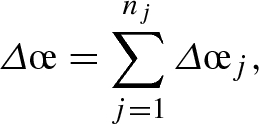(108)

where the subscript j indicates interaction with a discrete jet. Recognizing that each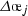is of the form: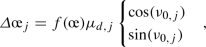(109)

where the cosine is used for $$\omega$$ and the sine is used for a and e. For simplicity, it can also be assumed that:

\begin{aligned} \rho _{0,j}=\frac{1}{n_j}\rho _0 , \end{aligned}
(110)

where the total production rate of the coma is divided evenly between the jets. It can also be assumed that these jets have some variation with latitude and longitude of the form $$\left[ (1-\alpha _{coma})+\alpha _{coma}\mathrm {f}(\theta _{j},\delta _{j}) \right]$$, consistent with the skewed coma model. If each of the jets has the same skew function and skewedness, this results in: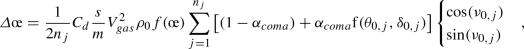(111)

where $$\theta _{0,j}$$ and $$\delta _{0,j}$$ are the latitude and longitude at the time of the $$j^{th}$$ jet crossing. Taking the limit as $$n_j \rightarrow \infty$$ the summation can be replaced with an integral over $$\rho _0$$, which can be converted to an integral over true anomaly: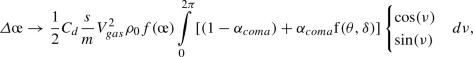(112)

which is equivalent to integrating Gauss’ equations for an arbitrary skew function.

## Comparison with numerical results

In this section, the differential equations for the mean elements are compared with numerical simulations. First, (Sect. 5.1) simulations using an arbitrary inverse-square radial perturbing acceleration (in the form of Eq. (31)) are compared to the semi-analytical results from integrating Eqs. (49) to (52). Second, (Sect. 5.2) the rotation-dependent coma and cannonball drag models, Eqs. (9) and (15), respectively, are used for numerical simulation. These results are compared with the semi-analytic solution for the mean elements (Eqs. (49) to (52)) using the Fourier coefficients for the rotation-dependent coma model (Eqs. (91) to (94)). Trajectories neglecting the spacecraft velocity in the drag calculation (Eq. (17)) are shown to illustrate the effect of neglecting the in-track component of the perturbing acceleration when propagating the spacecraft state as opposed to the mean elements. Strengths and weaknesses of the semi-analytic approach are discussed in the context of the approximations used to derive those results.

The equations of motion, Eq. (18), are integrated in inertial Cartesian coordinates using a variable timestep RK45 integrator, specifically Matlab’s ode45. Osculating orbital elements are calculated from the Cartesian state using the perturbed gravitational parameter derived in Eq. (48) at each timestep. The semi-analytical solution is found by numerically integrating the differential equations for the mean elements (Eqs. (49) to (52)). The initial conditions for the mean elements are found by back-propagating the numerical mean elements at a time of two Keplerian orbital periods, calculated using the initial semi-major axis, to the initial time. The numerical mean elements are calculated using the instantaneous Keplerian orbital period at the time of interest. The osculating elements are integrated, using the trapezoidal approximation, over a one period wide window centered on the time at which the average is being calculated. The numerical average is thus the trapezoidal integral divided by the period of integration.

All numerical simulations are roughly based on comet 67P/Churyumov–Gerasimenko, the target of the Rosetta mission and assume an unperturbed gravitational parameter of 665 $$m^3/s^2$$ (Pätzold et al. 2018). As discussed in Sect. 3, the dynamics used in this paper are a valid approximation for distances of a few nuclear radii to $$\sim 1/2$$ the Hill radius from the comet.

### Purely radial Fourier series model

Propagation of the Cartesian state using the purely radial Fourier series model (Eq. (31)) compares well with the semi-analytic solution for the mean elements. Recall that the purely radial Fourier series model is the exact model used to derive the semi-analytic results. Figure 2 is an example trajectory where the Fourier coefficients were randomly generated up to order 19 (see Table 1 for values). Note that this perturbing acceleration is not strictly radially outward, which is unrealistic for the coma drag perturbation, but demonstrates the generality of the analysis. Furthermore, the solution structure is unaffected by the sign of the perturbing acceleration, governed by $$A_0$$. Only the timescale of the orbit is affected by $$A_0$$.

The semi-analytical results closely track the Cartesian propagation, though these do not match exactly due to the fact that the numerically calculated mean elements are not exactly the mean elements. These errors result in imperfect corrected initial conditions. The argument of crossing does not depend on the orbital elements and is accurately determined by Eq. (67). This particular simulation begins with the argument of pericenter near the unstable equilibrium, thus shrinking the semi-major axis and eccentricity. The argument of pericenter then migrates toward the stable equilibrium, driving the semi-major axis and eccentricity up. Should the simulation have been run longer, the spacecraft would escape the system. Note that escape can only be approached by propagating the mean elements and is thus not predicted by the semi-analytic solution. Escape comes about due to the osculation about the average and is thus consistent with the analysis presented above. One can investigate the magnitude of the short-period variations superimposed on the long-term trends described by the semi-analytic solution to predict escape, but this is beyond the scope of this paper.

### Full drag dynamics

In this subsection, the full drag dynamics described by Eq. (15) and the rotation-dependent coma model described in Sect. 2.1.3 are used for propagating trajectories. For comparison, trajectories using the simplified, purely radial, drag model (Eq. (17)) are also shown. The semi-analytic solutions for the mean elements (Eqs. (49) to (52)) are also computed using the Fourier coefficients given by Eqs. (91) to (94). The quality and limits of the purely radial assumption used to simplify the dynamics to derive analytical solutions are demonstrated. In each case, the simulated spacecraft is assumed to be Rosetta-like in size and mass. A mass of 2000 kg, an area of 70 $$m^2$$, and a drag coefficient of 2.2 are used. Note that the cannonball drag approximation may not be well suited for spacecraft with large, planar, solar panels; however, the ballistic coefficient (area-to-mass ratio) of the simulated spacecraft is comparable to that of centimeter to decimeter sized “boulders” of cometary material. A gas speed of 300 m/s was chosen to be on the low end of realistic values to maximize the effect of including the relative velocity of the spacecraft in the simulations. Table 2 contains the other relevant simulation constants used in Figs. 3, 4, 5, and 6.

Figure 3 shows a trajectory initialized near the unstable equilibrium argument of pericenter. The coma has a skewedness of 1; therefore, the full drag dynamics will be as close to the simplified, purely radial, dynamics as possible. The trajectories propagated with the purely radial and full drag dynamics track each other closely and are well reproduced by the semi-analytic solution for the mean elements, validating the assumption that the velocity of the spacecraft can be neglected for the averaging analysis. Note how sharply the argument of pericenter migrates from the unstable to stable equilibrium. Inspecting the Cartesian representations of the orbits propagated with the full drag model and the purely radial drag model, the slow damping of energy from the system through the in-track component of the drag can be seen; however, it is not obvious from the osculating and mean elements. The perturbation effects due to the skewed component of the coma have much shorter timescales than the analytically unmodeled, in-track, drag acceleration and thus dominate.

The simulations shown in Fig. 4 use the same parameters as in Fig. 3; however, this orbit is in the terminator plane, with an inclination just below 90$$^\circ$$ to allow evaluation of the elliptic integral in Eq. (92) as $$\tan (i)$$ goes to infinity. On average, the orbital elements should be constant as the first-order Fourier coefficients $$A_1$$ and $$B_1$$ are zero (Eqs. (91)–(94)), and in-fact the trajectory propagated with the purely radial drag model is repeating. The trajectory propagated with the full drag dynamics is slowly decaying and circularizing, as one would expect from a drag acceleration with an in-track component, though this is ignored in the analytical analysis.

One can also ask how the skewedness effects trajectories propagated with the full drag dynamics. Trajectories in Figs. 5 and 6 were propagated using comae that are much denser and less skewed (lower $$\alpha _{coma}$$) than those used in previous simulations to investigate this effect. Figure 5 replicates Fig. 3, where the initial argument of pericenter is near the unstable equilibrium. It is telling that even with a low skewedness of  0.05, the skew effects dominate over the in-track drag effects. The trajectories in Fig. 6 begin with near zero eccentricity and have a randomly chosen inclination and right ascension of the ascending node. Though the integrated trajectories look significantly different for each set of dynamics, the semi-analytic solution for the mean elements tracks the osculating elements well. The effects of the in-track component of drag are most evident in the semi-major axis plots, where the osculating elements trend lower than the semi-analytical solution for the mean elements as time goes on and more energy is damped from the system, though the effect is small. This indicates that the orbit-averaging analysis is valid for small deviations from spherical symmetry where the assumptions of the analysis begin to breakdown.